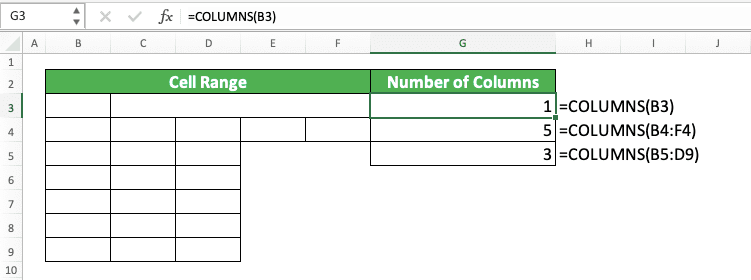How to Use the COLUMNS Formula in Excel: Functions, Examples, and Writing Steps - Compute Expert

# How to Use the COLUMNS Formula in Excel: Functions, Examples, and Writing Steps

Home >> Excel Tutorials from Compute Expert >> Excel Formulas List >> How to Use the COLUMNS Formula in Excel: Functions, Examples, and Writing Steps

In this tutorial, you will learn how to use the COLUMNS formula in excel completely.

When working in excel, we might sometimes want to know the number of columns that our cell range has. If we know how to use COLUMNS in excel, then we can get that number of columns easily.

Want to know more about this COLUMNS function and how to use it properly in excel? Read this tutorial until its last part!

Disclaimer: This post may contain affiliate links from which we earn commission from qualifying purchases/actions at no additional cost for you. Learn more

## What is the COLUMNS Formula in Excel?

COLUMNS formula in excel is a formula that helps you to get the number of columns in a cell range.

## COLUMNS Function in Excel

You can use COLUMNS to count the number of columns in the cell range you input into it.

## COLUMNS Result

The COLUMNS result is a number that represents the number of columns in the cell range you input into COLUMNS.

## Excel Version from Which We Can Start Using COLUMNS

We can start using COLUMNS in excel since excel 2003.

## The Way to Write It and Its Input

Here is the general writing form of COLUMNS in excel.

= COLUMNS ( cell_range )

You only need to input the cell range you want to get the number of columns of when you write COLUMNS.

## Example of Its Usage and Input

Here is a usage and result example of the COLUMNS function in excel.As you can see in the example, the way to use COLUMNS is quite easy. Just input the cell range you want to count the number of columns of and press enter.

By doing that, you will immediately get the number of columns in that cell range you input.

## Writing Steps

Want guidance when you write your COLUMNS formula in excel? Read these COLUMNS writing steps we have created for you below!

1. Type an equal sign ( = ) in the cell where you want to put the COLUMNS result2. Type COLUMNS (can be with large and small letters) and an open bracket sign after =3. Input the cell range that you want to get the number of columns of4. Type a close bracket sign5. Press Enter
6. Done!## Count the Number of Cells in a Cell Range: COLUMNS ROWS

What if instead of counting the number of columns, you want to count the number of cells in your cell range? If that is what you need, you can combine COLUMNS and ROWS functions for that.

COLUMNS will get you the cell range’s number of columns while ROWS will get you the cell range’s number of rows. If you multiply their results, you will get the number of cells in your cell range.

Therefore, here is the general writing form of the COLUMNS and ROWS combination in excel.

= COLUMNS ( cell_range ) * ROWS ( cell_range )

Just put the star symbol ( * ) between your COLUMNS and ROWS functions and you are good to go! Input the cell range you want to get the number of cells of in the COLUMNS and ROWS you write.

Here is the implementation example of the COLUMNS and ROWS combination in excel.As you can see there, we can get the number of cells of our cell range by combining COLUMNS and ROWS. Just input the cell range to COLUMNS and ROWS and multiply their results to immediately get the number of cells.

## Exercise

After you have learned how to use COLUMNS in excel, let’s do this exercise to sharpen your understanding!

### Instructions

Answer each question below in the appropriate gray-colored cell according to the question number. Give all your answers by using the excel COLUMNS function.
1. How many columns does the yellow cell range have?
2. How many columns does the blue cell range have?
3. How many cells does the blue cell range have?

You can also get the number of members that an array has in excel by using COLUMNS. For example, if you write this in your cell.

= COLUMNS ( { “a” , 1 , 2 , 3 , “b” } )

Then you will get 5 as the COLUMNS result.

Related tutorials you should learn too:

Get updated excel info from Compute Expert by registering your email. It's free!